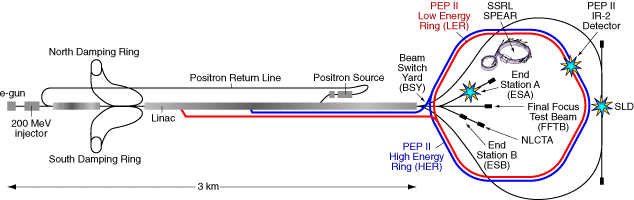# Physics 141, Fall 2016.## Physics 141, Homework Set # 04. Due: 10/7/16, 12 pm (noon).

1.     (50%) WeBWorK set # 4.

2.     (25%) The Stanford Linear Accelerator Center (SLAC), located at Stanford University in Palo Alto, California, accelerates electrons through a vacuum tube, 3-km long.Electrons, which are initially at rest, are subjected to a continuous force of 2 x 10-12 N along the entire 3-km length of the tube and reach speeds very close to the speed of light.  Using the material discussed in Chapter 5,

a.     Calculate the final energy, momentum, and speed of the electron.

b.     Calculate the time required to go the 3-km distance.

3.     (25%) A particle with mass M and charge +e and its antiparticle (same mass M, charge -e) are initially at rest, far away from each other.  The attract each other and move toward each other.

a.     Make a graph of the dependence of the various energies that are involved in this process (the potential energy, the particle energies - including the rest energy -, and the total energy) as function of distance.

b.     When the particle and antiparticle collide, they annihilate and produce a different particle with rest mass m (which is much smaller than M) and charge +e and its antiparticle (same rest mass m, charge -e).  When these particles have moved far away from each other, how fast are they going?  Is this speed large or small compared to the speed of light?

c.     Now take the specific case of a proton and antiproton colliding to form a positive and negative pion.  Each pion has a rest mass of 2.5 x 10-28 kg.  When the pions have moved far away, how fast are they going?

4.     (Optional; 25% extra credit) Write a program in VPython to determine the final speed of each electron, and the time required to cover the length of the tube.  You can use the VPython program classicalMotion.py from the Physics 141 software area as a starting point.  However, since the electrons will move with velocities very close to the speed of light, you can no longer assume that v = p/m and you have to make sure you use the relativistically correct equations to simulate the motion.  Submit the actual program via email to Professor Wolfs (wolfs@pas.rochester.edu).  The name of the file should be hw04p04XXYYYYYYYY.py where XX are your initials and YYYYYYYY is your student id number.  Compare you answer to this simulation with the answer you obtained in Problem 2 of this assignment.

5.     (Optional; 25% extra credit) Create a computer model of a metal bar of length L by considering N atoms of mass m connected by interatomic springs in a straight line.  You can use the VPython program modelMetalBar.py from the Physics 141 software area as a starting point.  Give the first atom a sudden push and compare the motions of al the atoms, one time step after another.  Calculate all of the forces before using these forces to update the momenta and positions of the atoms.  After each time step, display the displacements of all the atoms away from their equilibrium positions, and determine the speed of sound (the distance between the first and last atom of the bar divided by the time it takes for the last atom to feel a non-zero force).  Compare your results with the prediction that the speed of sound iswhere D is L/N and k is the spring constant.  How does the agreement change when you change the number of atoms in your model?  Submit your answers and your actual program via email to Professor Wolfs (wolfs@pas.rochester.edu).  The name of the file should be hw04p05XXYYYYYYYY.py where XX are your initials and YYYYYYYY is your student id number.

Last updated on Monday, September 26, 2016 8:41

Instructor Home Page | Instructor Contact Information | Email the Instructor | Home | © 2016 University of Rochester